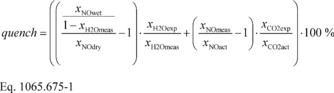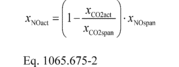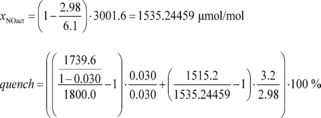# Title 40

## SECTION 1065.675

### 1065.675 CLD quench verification calculations.

§ 1065.675 CLD quench verification calculations.

Perform CLD quench-check calculations as follows:

(a) Perform a CLD analyzer quench verification test as described in § 1065.370.

(b) Estimate the maximum expected mole fraction of water during emission testing, xH2Oexp. Make this estimate where the humidified NO span gas was introduced in § 1065.370(e)(6). When estimating the maximum expected mole fraction of water, consider the maximum expected water content in combustion air, fuel combustion products, and dilution air (if applicable). If you introduced the humidified NO span gas into the sample system upstream of a sample dryer during the verification test, you need not estimate the maximum expected mole fraction of water and you must set xH2Oexp equal to xH2Omeas.

(c) Estimate the maximum expected CO2 concentration during emission testing, xCO2exp. Make this estimate at the sample system location where the blended NO and CO2 span gases are introduced according to § 1065.370(d)(10). When estimating the maximum expected CO2 concentration, consider the maximum expected CO2 content in fuel combustion products and dilution air.

(d) Calculate quench as follows:Where: quench = amount of CLD quench. xNOdry = concentration of NO upstream of a bubbler, according to § 1065.370(e)(4). xNOwet = measured concentration of NO downstream of a bubbler, according to § 1065.370(e)(9). xH2Oexp = maximum expected mole fraction of water during emission testing, according to paragraph (b) of this section. xH2Omeas = measured mole fraction of water during the quench verification, according to § 1065.370(e)(7). xNOmeas = measured concentration of NO when NO span gas is blended with CO2 span gas, according to § 1065.370(d)(10). xNOact = actual concentration of NO when NO span gas is blended with CO2 span gas, according to § 1065.370(d)(11) and calculated according to Eq. 1065.675-2. xCO2exp = maximum expected concentration of CO2 during emission testing, according to paragraph (c) of this section. xCO2act = actual concentration of CO2 when NO span gas is blended with CO2 span gas, according to § 1065.370(d)(9).Where: xNOspan = The NO span gas concentration input to the gas divider, according to § 1065.370(d)(5). xCO2span = the CO2 span gas concentration input to the gas divider, according to § 1065.370(d)(4). Example: xNOdry = 1800.0 µmol/mol xNOwet = 1739.6 µmol/mol xH2Oexp = 0.030 mol/mol xH2Omeas = 0.030 mol/mol xNOmeas = 1515.2 µmol/mol xNOspan = 3001.6 µmol/mol xCO2exp = 3.2% xCO2span = 6.1% xCO2act = 2.98%quench = (−0.0036655−0.014020171)·100% = −1.7685671% [73 FR 59340, Oct. 8, 2008, as amended at 76 FR 57466, Sept. 15, 2011; 81 FR 74188, Oct. 25, 2016]